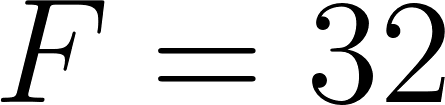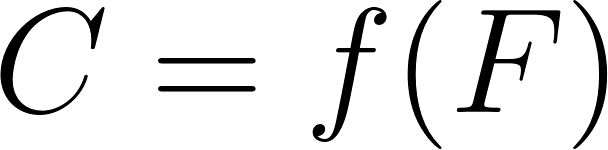×
Get Full Access to Calculus: Early Transcendentals - 1 Edition - Chapter 1.2 - Problem 56e
Get Full Access to Calculus: Early Transcendentals - 1 Edition - Chapter 1.2 - Problem 56e

×

# Temperature scales a. Find the linear function C = f )ISBN: 9780321570567 2

## Solution for problem 56E Chapter 1.2

Calculus: Early Transcendentals | 1st Edition

• Textbook Solutions
• 2901 Step-by-step solutions solved by professors and subject experts
• Get 24/7 help from StudySoup virtual teaching assistantsCalculus: Early Transcendentals | 1st Edition

4 5 1 379 Reviews
22
3
Problem 56E

Temperature scales:

a. Find the linear functionthat gives the reading on the Celsius temperature scale corresponding to a reading on the Fahrenheit scale. Use the facts thatwhen(freezing point) andwhen(boiling point).

b. At what temperature are the Celsius and Fahrenheit readings equal?

Step-by-Step Solution:

Step 1 of 4

Given facts,whenwhenWe need to find the linear functionStep 2 of 4

Step 3 of 4

##### ISBN: 9780321570567

The full step-by-step solution to problem: 56E from chapter: 1.2 was answered by , our top Calculus solution expert on 03/03/17, 03:45PM. The answer to “?Temperature scales: a. Find the linear function that gives the reading on the Celsius temperature scale corresponding to a reading on the Fahrenheit scale. Use the facts that when (freezing point) and when (boiling point). b. At what temperature are the Celsius and Fahrenheit readings equal?” is broken down into a number of easy to follow steps, and 46 words. Since the solution to 56E from 1.2 chapter was answered, more than 391 students have viewed the full step-by-step answer. This full solution covers the following key subjects: temperature, Reading, Scale, celsius, point. This expansive textbook survival guide covers 85 chapters, and 5218 solutions. Calculus: Early Transcendentals was written by and is associated to the ISBN: 9780321570567. This textbook survival guide was created for the textbook: Calculus: Early Transcendentals, edition: 1.

Unlock Textbook Solution